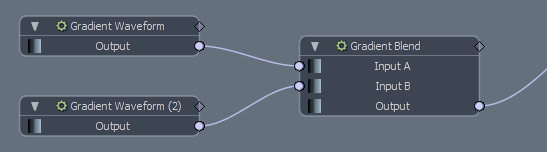## Example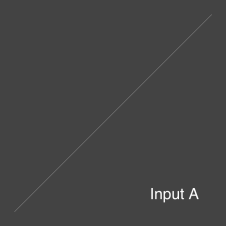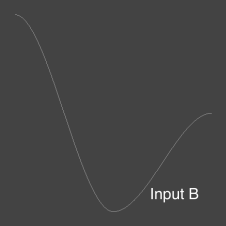Input A is a linear gradient. Input B is a curved gradient.

With a blend amount of 0%, the linear gradient is the output. With a blend amount of 100%, the curved gradient is the output. If the blend amount is 50%, the output is a mix of the linear and the curved gradient.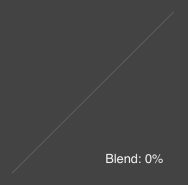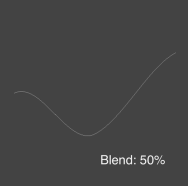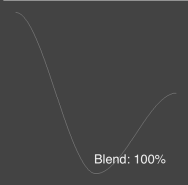Channels on the Node by Default Input A The gradient to blend from. Input B The gradient to blend to. Output The blended gradient. Properties Panel Mode The type of value used for modulating the interpolation. The following options are available: Scalar - The default value. The blend is uniform along the length of the gradient, controlled by the Blend Amount channel. Gradient - The blend varies along the length of the gradient, controlled by the Blend Gradient channel. Blend When Mode is set to Scalar, specifies the amount to blend between Input A and Input B.

The Gradient Clamp modifier clamps the gradient output value, ensuring that the value never exceeds the specified minimum and maximum range.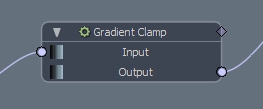## Example

The following images show a gradient clamped at a Minimum Value of 0.25 and a Maximum value of 0.75.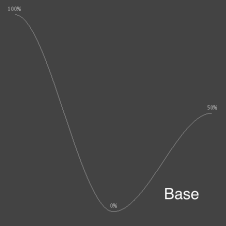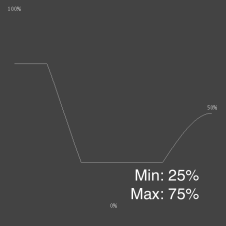The input gradient. The clamped gradient.

 Channels on the Node by Default Input The gradient to clamp. Output The clamped gradient. Properties Panel Minimum Value The lower end of the clamped range. Maximum Value The upper end of the clamped range.

The Gradient from Mesh modifier generates a gradient from a mesh layer. It maps the height of the mesh in either the X, Y, or Z axis to the value of the gradient.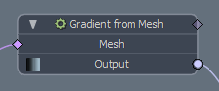The modifier takes a slice through the input mesh, and raycasts into the slice to extract the height of the surface. You can specify the axis and offset for the slice, as well as animate it, allowing the slope of the gradient to be modified over time.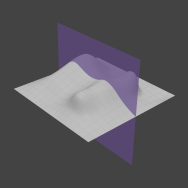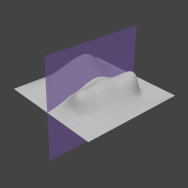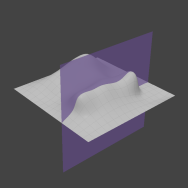Channels on the Node by Default Mesh The mesh used for producing the gradient. Output The computed gradient. Properties Panel Axis The axis along which the raycast is projected. The height of the mesh in the axis determines the value of the gradient. Slice Axis The axis along which the mesh is sliced. U and V maps to the two axes not specified as Axis for raycasting. For example, if the raycasting axis is Y, U and V in the Slice Axis represent X and Z respectively. Slice Offset The offset along the slice axis to slice the mesh. Animating this value moves the slicing plane forwards and backwards, animating the value of the gradient. Range Minimum/Range Maximum The bounding box of the mesh is read as the extremes of the gradient, but the way they map to gradient values is determined by the Range Minimum and Range Maximum options. Changing these values control how the smallest and largest extremes of the bounding box map to the smallest and largest time values in the gradient.

The Gradient Math modifier performs basic math operations on any number of gradient inputs.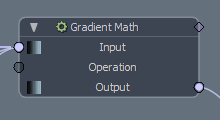## Example

Let's take these three input gradients: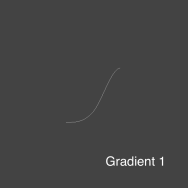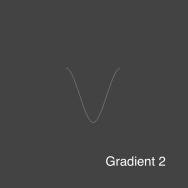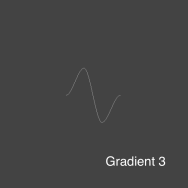The following results are generated using each math operation: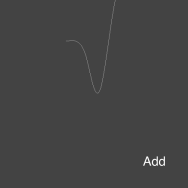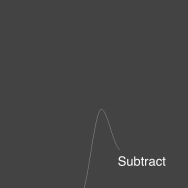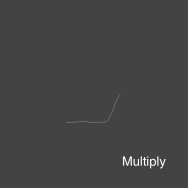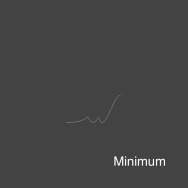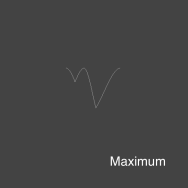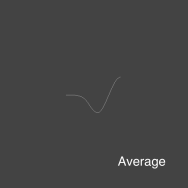The Gradient Noise modifier applies Perlin Noise to an existing gradient. The modifier provides control over both noise amplitude and frequency.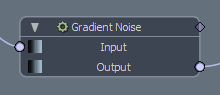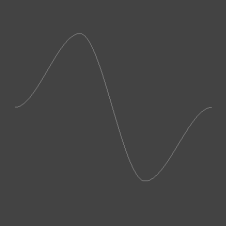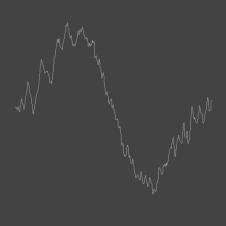The input gradient. The gradient with noise applied to it.

 Channels on the Node by Default Input The source gradient. Output The computed gradient with noise applied. Properties Panel Frequency Determines how regularly the noise occurs per second. Amplitude Controls how strong the noise offsets the original base gradient. Seed Seeds the randomness of the noise generator. Animating this value animates the noise.

The Gradient Offset node offsets all the values in a gradient equally, either by offsetting the value in Y, and/or the time the value is sampled in X.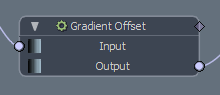## Example

This example shows the source gradient offset by 0.33 on X and by 0.33 on Y.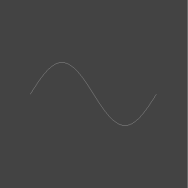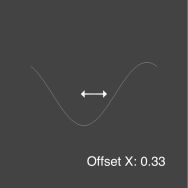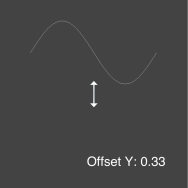Channels on the Node by Default Input The gradient to offset. Output The offset gradient. Properties Panel Offset X Offsets the gradient value in X. This offsets the sample time, so reading the gradient at a specific time sample remaps the time sample to read the input gradient at a different time. Offset Y Remaps the gradient value, adding the Offset Y value to the input gradient sampled value.

The Gradient Scale modifier scales a gradient either in the Y axis, modifying the gradient's value, or the X axis, modifying the time of each value. You can also control the pivot point for each axis the scaling is performed in.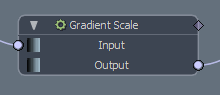## Example

This example shows the input gradient scaled on the X and then the Y axis by 0.25 and 2.0.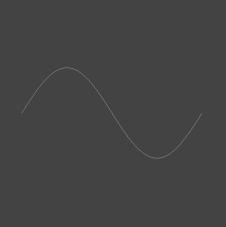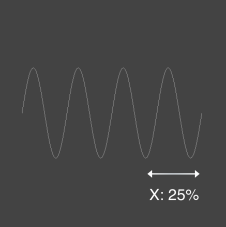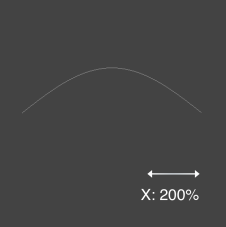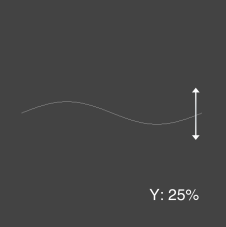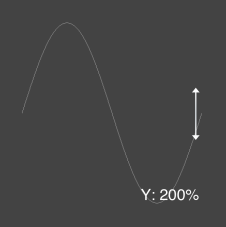Channels on the Node by Default Input The gradient to scale. Output The scaled gradient. Properties Panel Amount X/Y The amount the input gradient is scaled in the X/Y axis. Changing X modifies the sample time of each value in the gradient. Changing Y increases or decreases the gradient values. Center X/Y The pivot point to perform the scale in the X/Y axis. The gradient is scaled from this position.

The Gradient Step modifier rounds values in a floating point gradient to their nearest whole number. For example, 0.25 becomes 0.0, and 0.6 becomes 1.0. This can introduce a step pattern to gradients that cover a large range of floating point values.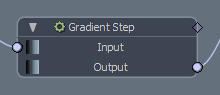## Example

In this example, the base gradient has a Maximum Value of 5.0 and a Minimum Value of -5.0. This introduces 11 steps across the range, as every value between -5.0 and +5.0 (including 0.0) is rounded to their nearest whole number.The source gradient. The output gradient.

 Channels on the Node by Default Input The input gradient. Output The stepped gradient.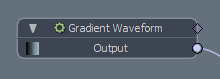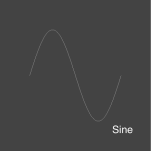A sinusoidal waveform that describes a smooth periodic oscillation from one extreme of the amplitude to the other.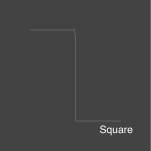A square wavefrom that rounds to the nearest amplitude value.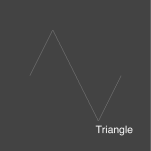A triangle waveform that blends in linearly from one extreme of the amplitude to the other.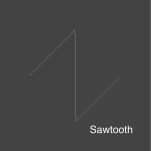A sawtooth waveform that blends linearly to the positive extreme of the amplitude, and then snaps back to the negative extreme of the amplitude.